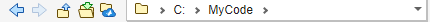# pwd

Identify current folder

## Syntax

``pwd``
``currentFolder = pwd``

## Description

``pwd` displays the MATLAB® current folder.`

example

````currentFolder = pwd` returns the path to the current folder.```

## Examples

### Store Path to MATLAB® Current Folder

Change the current folder to a local folder and store the path.

```cd c:\myMATLABFiles currentFolder = pwd ```
```currentFolder = 'c:\myMATLABFiles' ```

### Alternative Functionality

View the current folder in the Current Folder toolbar.## Output Arguments

collapse all

MATLAB current folder path, returned as a character vector.